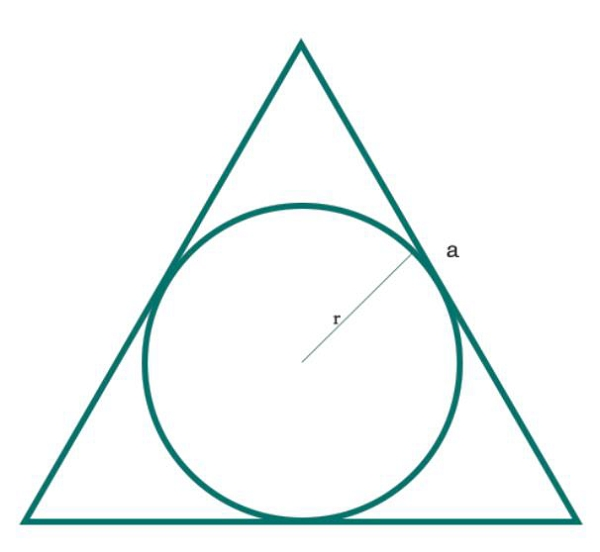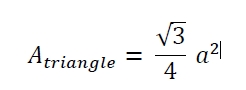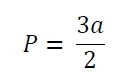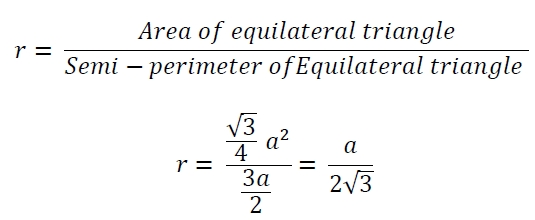# Area of circle which is inscribed in equilateral triangle in C Program?

Here we will see the area of circle which is inscribed in an equilateral triangle. The sides of the triangle are ‘a’.The area of equilateral triangle −The semi-perimeter of the triangle is −So the radius of the circle is −## Example

#include <iostream>
#include <cmath>
using namespace std;
float area(float a) {
if (a < 0 ) //if the value is negative it is invalid
return -1;
float area = 3.1415 * (a/(2*sqrt(3))) * (a/(2*sqrt(3)));
return area;
}
int main() {
float a = 4;
cout << "Area is: " << area(a);
}

## Output

Area is: 4.18867